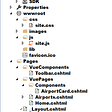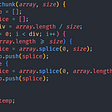# Depth First Search (Javascript)

`class Node {   constructor(val) {     this.val = val;     this.left = null;      this.right = null;  }}`
`const a = new Node (1)const b = new Node (2)const c = new Node (3)const d = new Node (4)const e = new Node (5)a.left = ba.right = cb.left = db.right = e        1       / \      2   3     / \    4   5      `
`const DFS = (root) => {   //make an empty array to house the root   let queue = [root]   //create an empty array to house the visited values   let stack = []   //create a while loop and the logic: "while the stack is greater than 0"   while (queue.length > 0) {          //create a variable = to the popped off iteration     let current = queue.pop()     //push the visited value into the stack          stack.push(current.val)     //loop through the left and right if there is a left and right      if (current.left) {       //if there is a left, push it into the stack        queue.push(current.left)      }     if (current.right) {queue.push(current.right)}}   return stack}`
`const DFS = (root) => {   //create your base case(s)   if (root === null) return []   //create your variables that recursively call the left and right   let left = DFS(root.left)   let right = DFS(root.right)    //use the spread operator to return an array of the current       //value, the left and the right values   return [root.val, ...left, ..right]}`

## More from Crystal Villanueva

Flatiron student, word nerd and otter enthusiast

Love podcasts or audiobooks? Learn on the go with our new app.

## How to Pass Props Through React-Router Path Links## P1 — From React Native to Flutter Web## D3 and React: Can’t We All Just Be Friends?## Handling Async Operations in Node.js## Create a SPA Vuejs Application in DotNetCore using a Razor Page and Vuejs Router without WebPack!## 🐞 Debugging NodeJS C++ addons using VS Code## One JS13k per day!## Crystal Villanueva

Flatiron student, word nerd and otter enthusiast

## Max Binary Heap in JavaScript## Deep Dive into Data structures using Javascript — Doubly Linked List## JavaScript Field Day — Logic is getting real.## Array chunk algorithm